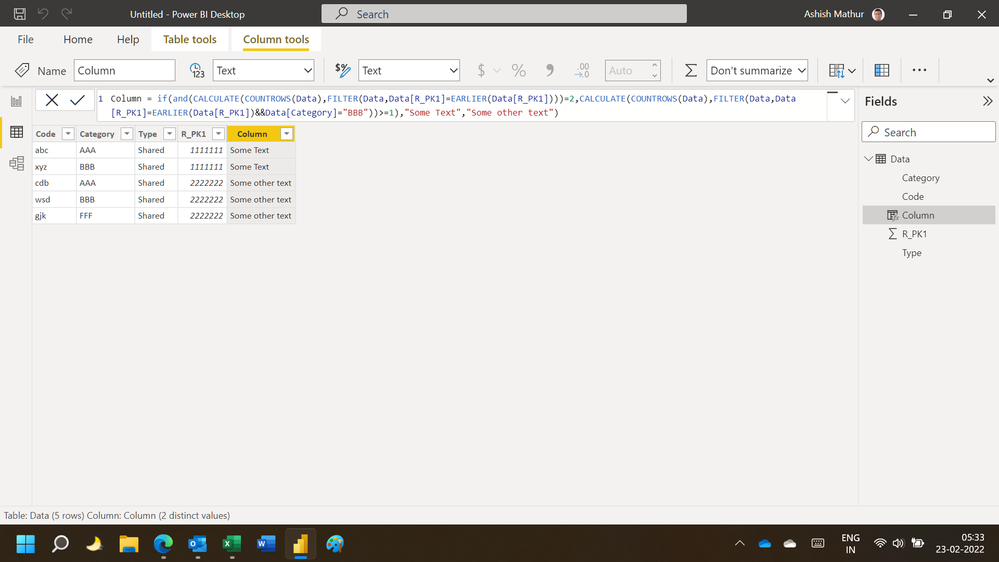cancel
Showing results for
Did you mean:Helper III

## Calculate if a column contains some text for each occurrence of a unique value

Hello,

I'm trying to come up with a formula for the following scenario. Any help would be greatly appreciated.

For a given Primary Key in column D ("R_PK1"), IF the Primary Key is present EXACTLY 2 times in the table, AND if column B equals "BBB" in any of the 2 rows for that Primary Key, then give me "some text" else "some other text".

Below is the sample data. Thank you for your help!

 Code: Category: Type: R_PK1: Output column: abc AAA Shared 1111111 some text xyz BBB Shared 1111111 some text cdb AAA Shared 2222222 some other text wsd BBB Shared 2222222 some other text gjk FFF Shared 2222222 some other text

2 ACCEPTED SOLUTIONSSuper User

Hi,

This calculated column formula works

``Column = if(and(CALCULATE(COUNTROWS(Data),FILTER(Data,Data[R_PK1]=EARLIER(Data[R_PK1])))=2,CALCULATE(COUNTROWS(Data),FILTER(Data,Data[R_PK1]=EARLIER(Data[R_PK1])&&Data[Category]="BBB"))>=1),"Some Text","Some other text")``

Hope this helps.Regards,
Ashish Mathur
http://www.ashishmathur.comCommunity Support

Hi  @tomekm ,

Here are the steps you can follow：

1. Create calculated column.

``````Output column =
var _count=COUNTX(FILTER(ALL('Table'),'Table'[R_PK1:]=EARLIER('Table'[R_PK1:])),[R_PK1:])
var _category=SELECTCOLUMNS(FILTER(ALL('Table'),[R_PK1:]=EARLIER('Table'[R_PK1:])),"1",'Table'[Category:])
return
IF(
_count=2&&"BBB" in _category,"Some Text","Some other text")``````

2. Result:Best Regards,

Liu Yang

If this post helps, then please consider Accept it as the solution to help the other members find it more quickly

7 REPLIES 7Community Support

Hi  @tomekm ,

Here are the steps you can follow：

1. Create calculated column.

``````Output column =
var _count=COUNTX(FILTER(ALL('Table'),'Table'[R_PK1:]=EARLIER('Table'[R_PK1:])),[R_PK1:])
var _category=SELECTCOLUMNS(FILTER(ALL('Table'),[R_PK1:]=EARLIER('Table'[R_PK1:])),"1",'Table'[Category:])
return
IF(
_count=2&&"BBB" in _category,"Some Text","Some other text")``````

2. Result:Best Regards,

Liu Yang

If this post helps, then please consider Accept it as the solution to help the other members find it more quicklySuper User

Hi,

This calculated column formula works

``Column = if(and(CALCULATE(COUNTROWS(Data),FILTER(Data,Data[R_PK1]=EARLIER(Data[R_PK1])))=2,CALCULATE(COUNTROWS(Data),FILTER(Data,Data[R_PK1]=EARLIER(Data[R_PK1])&&Data[Category]="BBB"))>=1),"Some Text","Some other text")``

Hope this helps.Regards,
Ashish Mathur
http://www.ashishmathur.comHelper III

Thank you!Super User

You are welcome.

Regards,
Ashish Mathur
http://www.ashishmathur.comSuper User

Hi @tomekm

Please try this, (change table name and col name as per quirement)
= VAR _count2 = DISTINCTCOUNT('Table'[R_PK1])
RESULT
SWITCH(
TRUE(),
_count2 = 2 && 'Table'[Category] = "BBB", "Some Test",
"Some Other Test")

If not solved, please keep posted (paste from excel, your data is broken when I try to use)

If this comment helps you, please LIKE this comment/KudosHelper III
 Code: Category: Type: R_PK1: Output column: abc AAA Shared 1111111 some text xyz BBB Shared 1111111 some text cdb AAA Shared 2222222 some other text wsd CCC Shared 2222222 some other text gjk FFF Shared 2222222 some other textHelper III

Thank you for the quick response. Unfortunately this formula didn't work for me. I'm pasting the data in tabular format here:

 Code: Category: Type: R_PK1: Output column: abc AAA Shared 1111111 some text xyz BBB Shared 1111111 some text cdb AAA Shared 2222222 some other text wsd CCC Shared 2222222 some other text gjk FFF Shared 2222222 some other text